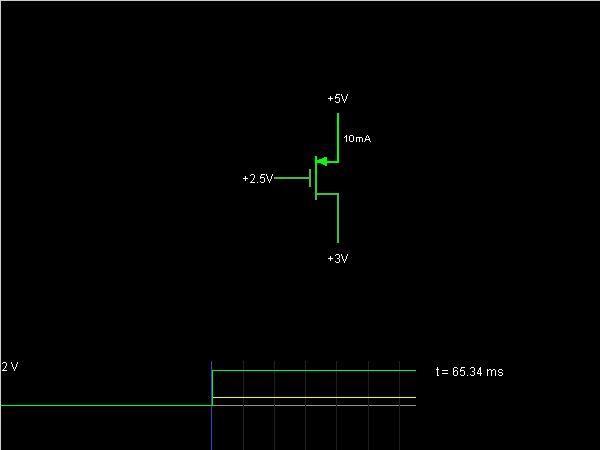# Circuit Simulator - p-MOSFET

## Where can I get p-MOSFET Circuit Diagram with Explanation?

IndiaBIX provides you lots of fully solved p-MOSFET circuit diagram with detailed explanation and working principles.

## How to design a p-MOSFET (electronic circuit)?

You can easily design the p-MOSFET circuit by practicing the exercises given below. Here you can design and simulate your own electronic circuits with this Online Circuit Designer and Simulator.### Circuit Description:

This is a simple model of a p-type MOSFET. The source is at 5 V, and the gate and drain voltages can be controlled using the sliders at the right.

Basically no current flows unless the gate voltage is lower than the source voltage by at least 1.5 V. (Threshold = -1.5 V) So if you have the gate lower than 3.5 V, current flows; otherwise not.

When the gate and drain voltages are sufficiently low, the MOSFET is in saturation, and the current is constant regardless of the drain voltage. If you raise the drain voltage enough relative to the gate, then the MOSFET is in linear mode, and the current is roughly linear to the source-drain voltage difference. By sliding the drain voltage slider slowly back and forth, you should be able to see the boundary between the linear and saturation regions. Try this with different settings for the gate voltage. -- Credits: Mr. Paul Falstad.